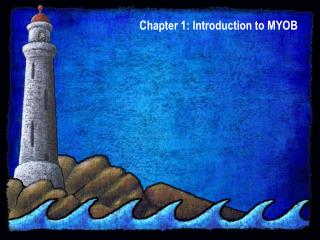DownloadDownload PresentationChapter 1: Introduction to MYOB

# Chapter 1: Introduction to MYOB

Download Presentation## Chapter 1: Introduction to MYOB

- - - - - - - - - - - - - - - - - - - - - - - - - - - E N D - - - - - - - - - - - - - - - - - - - - - - - - - - -
##### Presentation Transcript

1. Chapter 1: Introduction to MYOB Chapter 1: Introduction to MYOB

2. Figure 1.1: The Accounts command centre options Chapter 1: Introduction to MYOB

3. Figure 1.2: An example of a To Do List for accounts payable Chapter 1: Introduction to MYOB

4. Figure 1.3: An example of using Find Transactions on a general ledger account Chapter 1: Introduction to MYOB

5. Figure 1.4: The Setup drop-down menu Chapter 1: Introduction to MYOB

6. Figure 1.5: The List drop-down menu and Payroll Categories sub-menu Chapter 1: Introduction to MYOB

7. Figure 1.6: Selections in a Report Customisation window Chapter 1: Introduction to MYOB

8. Figure 1.7: Security Preferences window Chapter 1: Introduction to MYOB

9. Figure 1.8: System Preferences window Chapter 1: Introduction to MYOB

10. Figure 1.9: Preferences window for Reports and Forms Chapter 1: Introduction to MYOB

11. Figure 1.10: Windows Preferences Chapter 1: Introduction to MYOB

12. Figure 1.11: Selecting a Purchase Invoice from the Transaction Journal DetailArrow Chapter 1: Introduction to MYOB

13. Figure 1.12: List of Quotes in the Purchases Register Chapter 1: Introduction to MYOB

14. Figure 1.13: Quote received from Marbuk Imports Chapter 1: Introduction to MYOB

15. Figure 1.14: The Company Information Window Chapter 1: Introduction to MYOB

16. Figure 1.15: Bank details for electronic payments Chapter 1: Introduction to MYOB

17. Figure 1.16: Selections in the BAS Information window Chapter 1: Introduction to MYOB

18. Figure 1.17: General journal entry Chapter 1: Introduction to MYOB

19. Figure 1.18: Recap Transaction window for a general journal entry Chapter 1: Introduction to MYOB

20. Figure 1.19: Item selected from a list Chapter 1: Introduction to MYOB

21. Figure 1.20: A Bill for the purchase of items on credit Chapter 1: Introduction to MYOB

22. Figure 1.21: Recap Transaction window for an item purchase Chapter 1: Introduction to MYOB

23. Figure 1.22: A new sale invoice for items sold on credit Chapter 1: Introduction to MYOB

24. Figure 1.23: Recap Transaction window for sale of inventory items Chapter 1: Introduction to MYOB

25. Figure 1.24: Payment received from a customer (put into Undeposited Funds) Chapter 1: Introduction to MYOB

26. Figure 1.25: Cheque received now deposited at bank Chapter 1: Introduction to MYOB

27. Figure 1.26: Payment to a supplier Chapter 1: Introduction to MYOB

28. Figure 1.27: Cheque used to pay electricity account Chapter 1: Introduction to MYOB

29. Figure 1.28: Standard Balance Sheet selected as the report to print Chapter 1: Introduction to MYOB

30. Figure 1.29: Report Customisation window for Standard Balance Sheet printout Chapter 1: Introduction to MYOB

31. Figure 1.30: Periodic v perpetual inventory systems - retailer Chapter 1: Introduction to MYOB

32. Figure 1.31: Recording an invoice in an integrated system INVOICE Account Receivable Inventory Record Journal Entries for GL Dr Accounts Receivable Control Cr Sales (Selling price on Invoice) Dr Cost of Goods Sold Cr Inventory (Cost price on inventory record) Chapter 1: Introduction to MYOB

33. Figure 1.32:Manual steps in accounts receivable/inventory system General Ledger Sales Journal Acc.Rec.Ledger Order Invoice GeneralLedger Stock Journal Stock Ledger Chapter 1: Introduction to MYOB

34. Figure 1.33:Manual steps in computerised accounts receivable/inventory system Enquiries & Reports Enter Order (computer operations) Chapter 1: Introduction to MYOB

35. Figure 1.34:Module integration in an accounting package Cash Receipts and payments Payroll Sales & Receivables Purchases & Payables General Ledger Accounts Rec. Ledger Accounts Pay.Ledger Inventory Records Chapter 1: Introduction to MYOB